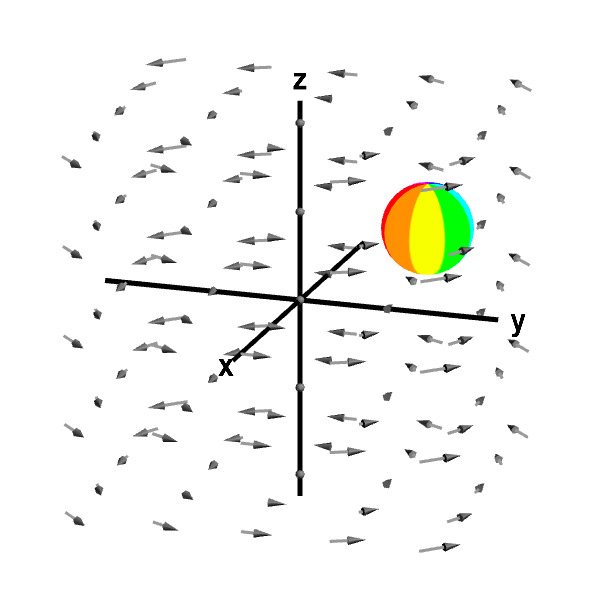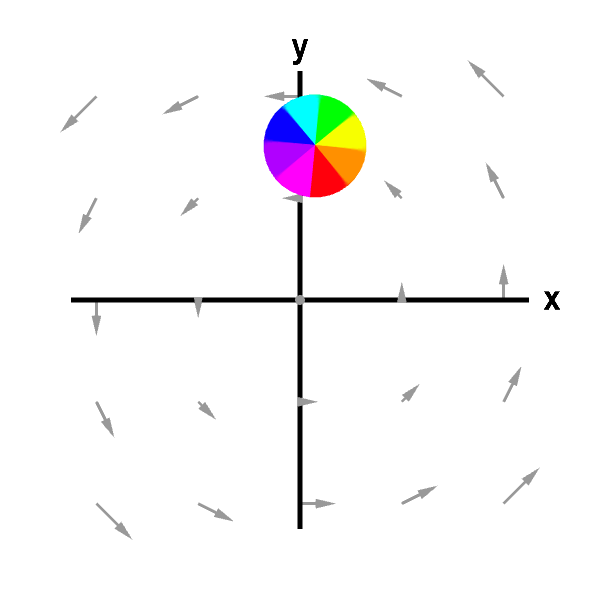# Math Insight

### Applet: Circling sphere in rotating vector fieldThe circling motion of the sphere is evidence of macroscopic circulation of the vector field (though not all macroscopic circulation would give a sphere circular motion). First panel shows the full vector field; second panel shows its projection in the $xy$-plane. You can rotate the view in the first panel with the mouse.

This vector field is $\dlvf(x,y,z)= (-y,x,0).$ Its curl is \begin{align*} \curl \dlvf(x,y,z) &= \left(\pdiff{}{y}0-\pdiff{}{z}x, \pdiff{}{z}(-y)- \pdiff{}{x}0, \pdiff{}{x}x - \pdiff{}{y}(-y) \right)\\ &=(0,0,2), \end{align*} which is a constant independent of point $(x,y,z)$ and always points in the positive $z$ direction.